# Fresnel Equations

(redirected from Fresnel equatations)

## Fresnel equations

[frā′nel i‚kwā·zhənz]
(optics)
Equations which give the intensity of each of the two polarization components of light which is reflected or transmitted at the boundary between two media with different indices of refraction.
McGraw-Hill Dictionary of Scientific & Technical Terms, 6E, Copyright © 2003 by The McGraw-Hill Companies, Inc.
The following article is from The Great Soviet Encyclopedia (1979). It might be outdated or ideologically biased.

## Fresnel Equations

formulas that relate the amplitude, phase, and state of polarization of reflected and refracted light waves that arise when light passes through a surface boundary between two transparent dielectrics to the corresponding characteristics of the incident wave. The equations were established by A. J. Fresnel in 1823 on the basis of conceptions about the elastic transverse vibrations of the ether. However, they can also be derived from the electromagnetic theory of light in the solution of Maxwell’s equations and the identification of the oscillations of light with oscillations of the electric field intensity in a light wave, with which most effects of wave optics are connected (seeMAXWELL’S EQUATIONS and FIELD INTENSITY, ELECTRIC).

Let a plane light wave strike a boundary between two media having refractive indexes n1 and n2 (see Figure 1). The angles φ, φ′, and ϕ” are the angles of incidence, reflection, and refraction, respectively. In this case, n1 sin ϕ = n2 sin ϕ” (the law of refraction) and |ϕ| = |ϕ’| (the law of reflection). We will decompose the electric vector of the incident wave into a component with amplitude Ap, parallel to the plane of incidence, and a component with amplitude As, perpendicular to the plane of incidence. Let us similarly decompose the amplitudes of the reflected wave into the components Rp and Rs and those of the refracted wave into Dp and Ds. The Fresnel equations for these amplitudes have the form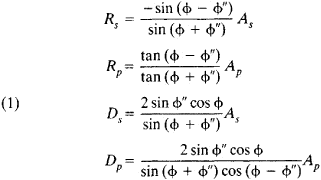It follows from equations (1) that for any value of the angles ϕ and ϕ” the signs of Ap and Dp and the signs of As and Ds coincide. This means that the phases also coincide; that is, in all cases the refracted wave retains the phase of the incident wave. For the components of the reflected wave (Rp and Rs), the phase relations depend on ϕ, n1, and n2. For example, if ϕ = 0, then when n2 > n1 the phase of the reflected wave will be shifted by π.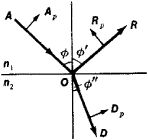Figure 1. Splitting of a ray of light incident on the boundary between two dielectrics into refracted ray D and reflected ray R. For simplicity, only the orientation of the p-components of these rays are shown, polarized parallel to the plane of incidence.

In experiments, rather than measuring the amplitude, scientists usually measure the intensity of a light wave, that is, the energy flux carried by it, which is proportional to the square of the amplitude (seePOYNTING VECTOR). The ratios of the average energy fluxes over a period of time in the reflected and refracted waves to the average energy flux in the incident wave are called the reflection coefficient r and the transmission coefficient d. We obtain from (1) the Fresnel equations that define the reflection and transmission coefficients for the s- and p-components of the incident wave:

(2) rs = (Rs/As)2
rp = (Rp/Ap)2
ds = (Ds/As)2
dp = (Dp/Ap)2

In the absence of light absorption, rs + ds = 1 and rp + dp = 1, in accordance with the law of the conservation of energy (seeABSORPTION OF LIGHT). If natural light is incident on the boundary (seePOLARIZATION OF LIGHT), that is, if all directions of oscillations of the electric vector are equally probable, then one-half of the wave’s energy is accounted for by p-oscillations and the other half by s-oscillations. In this case, the total reflection coefficient is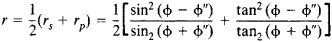If ϕ + ϕ” = 90° and tan (ϕ + ϕ”) → ∞, then rp = 0; that is, light that is polarized such that its electric vector lies in the plane of incidence under these conditions will not be reflected at all from the boundary. The reflected light, when natural light is incident at this angle, will be completely polarized. The angle of incidence at which this occurs is called the polarizing angle, or the Brewster angle (seeBREWSTER’S LAW). The relation tan ϕB = n2/n1 is valid for the Brewster angle.

For normal incidence of light on the boundary between two media (ϕ = 0), the Fresnel equations for the amplitudes of reflected and refracted waves can be reduced to the form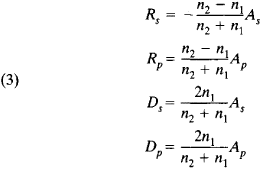In such a case, the difference between the components s and p disappears, since the concept of plane of incidence loses meaning. In this case, we obtain, in particular,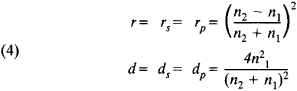It follows from (4) that the reflection of light at the boundary is greater the larger the absolute value of the difference n2 – n1; the coefficients r and d do not depend on which side of the boundary the incident light wave arrives from.

A condition for applicability of the Fresnel equations is that the refractive index of the medium be independent of the amplitude of the electric field strength of the light wave. This condition, which is trivial in classical (linear) optics, is not satisfied for high-power radiant fluxes, such as those radiated by lasers. In these cases, the Fresnel equations do not provide a satisfactory description of the observed phenomena, and consequently the methods and concepts of nonlinear optics must be used. (See alsoNONLINEAR OPTICS; REFLECTION OF LIGHT; OPTICS OF THIN FILMS; and REFRACTION OF LIGHT.)

### REFERENCES

Kaliteevskii, N. I. Volnovaia optika. Moscow, 1971.
Born, M., and E. Wolf. Osnovy optiki, 2nd ed. Moscow, 1973. (Translated from English.)
Landsberg, G. S. Optika, 5th ed. (Obshchii kurs fiziki.)

L. N. KAPORSKII